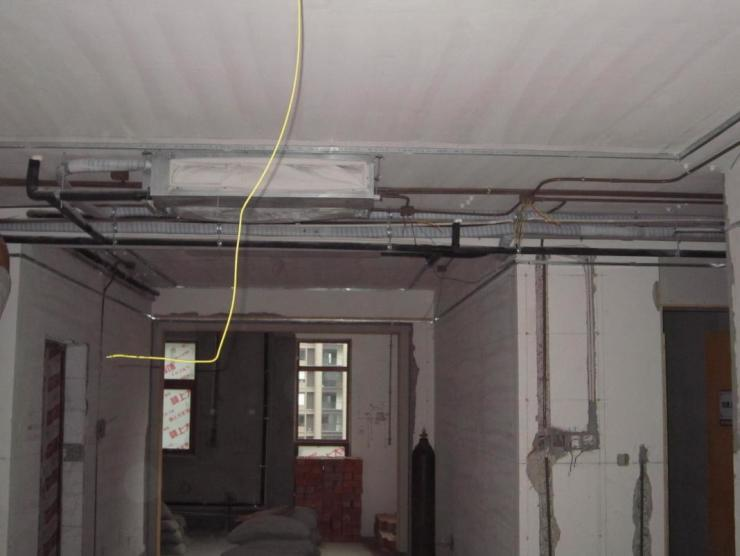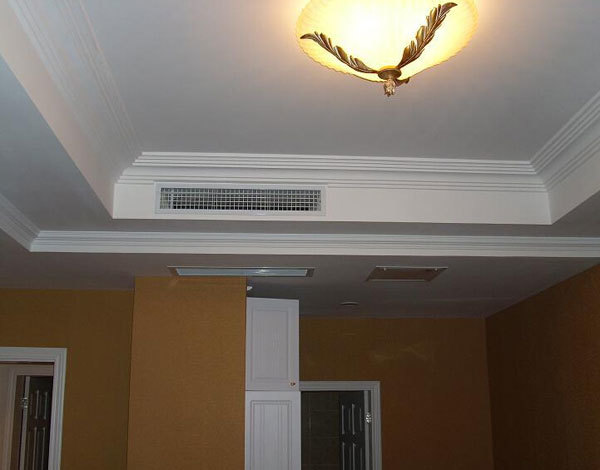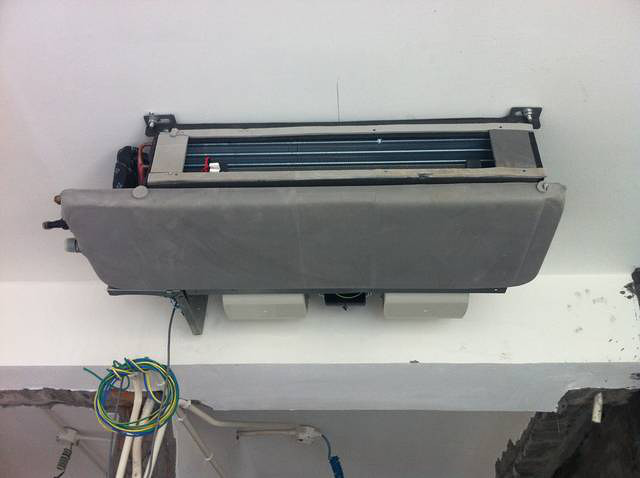|

# 什么是风管机 风管机和中央空调的区别

什么是风管机呢?风管机和中央空调的区别是什么呢?风管机安装方法是什么呢?一起来具体的看看吧~什么是风管机

风管机即风管式空调机，风管机是今年新兴的一种空调类型，它主要是通过联接风管向室内送风。相较于中央空调，风管机比较经济实惠，而相较于传统的柜式、挂式机，风管机的安装效果更美观。目前的风管机大多是1拖1的形式，用户在安装时可以根据自己的需求选择安装一台或多台。

风管机和中央空调的区别

风管机普遍是一拖一的配置，而中央空调是一拖多，只需要一台室外机，可以供应多个室内机一起工作，比较适合家用。风管机则比较适合餐厅、咖啡厅、商场等独立场所使用。

风管机和中央空调在使用效果上也有很大差别。中央空调出风均匀、柔和，不扬尘、不会污染室内空气，使用舒适度高。而且可以分室控制，并采用直流变频技术，非常节能。风管机安装方法

一、制作静压箱

根据用户需要的风口数量和室内机的出风口的尺寸，制作静压箱。如果用户需要3个出风口就做3个出风口的静压箱。静压箱用厚0.5～O.8mm的镀锌铁板做成。有几路风口就做几个风道口。风道口直径为200mm，高180mm，顶部外翻卷边，静压箱的进风口略大于室内机的出风口，箱体高400mm、宽300mm。静压箱的外形如右图所示。

二、制作风道接口

风道接头用0.5～O.8mm的镀锌铁板做成φ200mm×200mm的圆筒，两端外翻卷边。

三、凿风道洞

首先根据风口的数量，确定风道洞的大小，然后用电锤和二锤在墙上开风道洞。例如：三个风道则需要开200mmX800mm的墙洞，其他依此类推。在每个风道口的墙壁上再开一条放入绝缘套管的槽沟，深约50mm、宽20～30mm，用于接控制风口的开关连线和开关。

四、装配静压箱

将静压箱的进风口套在室内机的出风口外侧，用φ3.2mm的钻头钻孔，然后再用铆钉铆死。将整个静压箱紧紧地固定在室内机上，然后采用8mm厚的PE棉包住静压箱，做保温处理。先将PE棉放在静压箱的出风口上，用手压出一个出风口的印子，再用钢锯条锯出圆洞。用毛刷沾胶(如309胶、好帮手)涂在PE棉上，再将静压箱也涂上胶、凉一会，然后将PE棉粘在静压箱上，出风口从洞中穿过，要按实粘牢。PE棉的接头处，要用锡箔纸贴实。五、安装风道

将风道内层套在静压箱的风口上，再用并行的12号铁丝拧紧，用锡箔纸沿着一周圈贴实。再将石棉保温层及外边的保护层套到底部，用并行的12号铁丝拧紧，再用锡箔纸沿着一周贴实。晃动风道，锡箔纸不应有裂纹。依此法，将各个风道固定好。

六、安装室内机

根据室内机四个吊孔的距离，用铅笔在吊室内机的天花板上画出固定螺丝的位置。钻孔后，将准备好的膨胀螺丝(该螺丝是在φ8mm的膨胀螺丝上焊接一段长490mm的全丝螺栓，再带2个螺帽放入孔内)拧紧，然后把室内机的四角放在螺栓内将室内机吊起。室内机的安装必须保持水平，否则会造成排水不畅，容易漏水。

七、安装排水管

排水管采用3/4″的PVC管，管外侧套保温层。将等径接头(带螺丝)的两端涂上PVC管胶水，一端放在室内机上，另一端将涂胶后的PVC管插入固定，一直接到下水道。安装时，应注意排水管的高低，观察排水是否顺畅。

八、固定风道

用φ8m的膨胀螺丝焊上φ8m×690mm的钢筋固定在风道的两侧，然后将钢筋折弯，兜起风道。也可以在风道的两侧打膨胀螺丝，再用铁丝吊起风道。九、缝制风道接口袋

将厚帆布裁成560mm×300mm的长条，再缝制成圆筒。然后将风道接口袋，一折一折地包在风道接头上，用并行铁丝拧紧，再用锡箔纸贴好连接处。风道接头的另一端套在风道上，先将风道的内层套好，用铁丝拧紧，再用锡箔纸贴好连接处。

十、连接出风口(标准风口)

将帆布套在风口上，用铁丝拧紧，外面贴上锡箔纸。

十一、安装塞外机

根据室外机的大小做好安装支架，再用φ14m×l00mm的膨胀螺丝固定安装支架，然后将室外机固定在支架上。

十二、室内外机的连接

1.配管的连接将φ9.52mm和φ19.05mm的铜管穿上保温管，扩好喇叭口后，分别连接到室内外机的高低压管上，然后排空。各接口要严格检漏。

2.电气部分的连接380V电源线用截面积4mm2×4芯的电缆线，信号线使用φ0.75mm的铜芯线，每根线上必须穿上线号，做好标记，然后一一对应接好。各风口供电可以用截面积1.5mm2×2芯的软铜芯线，穿在蛇形绝缘套管里，一端接室内机，另_端接各风口。

3.风口开关的连接如左图所示，将截面积O.75mm2x3芯的电缆线穿在绝缘套管里，埋在各门口所开的线槽内，用于接控制风口的开关。将电缆一端的其中两根接控制开关的两个静触头，另一端的这2根线接风口电机的控制端。剩下的一根剪断，去开关的一根接主电源I二的火线，去风口的一根接主电源线上的零线。

最后，装修时需要留净空520mm×140mm的出风口，820mm×370mm的回气口，300mm×1500mm的检修口，检修口必须能够方便地拆下两个电机。

`声明：本文由入驻焦点开放平台的作者撰写，除焦点官方账号外，观点仅代表作者本人，不代表焦点立场错误信息举报电话： 400-099-0099，邮箱：jubao@vip.sohu.com，或点此进行意见反馈，或点此进行举报投诉。`A B C D E F G H J K L M N P Q R S T W X Y Z
A - B - C - D - E
• A
• 鞍山
• 安庆
• 安阳
• 安顺
• 安康
• 澳门
• B
• 北京
• 保定
• 包头
• 巴彦淖尔
• 本溪
• 蚌埠
• 亳州
• 滨州
• 北海
• 百色
• 巴中
• 毕节
• 保山
• 宝鸡
• 白银
• 巴州
• C
• 承德
• 沧州
• 长治
• 赤峰
• 朝阳
• 长春
• 常州
• 滁州
• 池州
• 长沙
• 常德
• 郴州
• 潮州
• 崇左
• 重庆
• 成都
• 楚雄
• 昌都
• 慈溪
• 常熟
• D
• 大同
• 大连
• 丹东
• 大庆
• 东营
• 德州
• 东莞
• 德阳
• 达州
• 大理
• 德宏
• 定西
• 儋州
• 东平
• E
• 鄂尔多斯
• 鄂州
• 恩施
F - G - H - I - J
• F
• 抚顺
• 阜新
• 阜阳
• 福州
• 抚州
• 佛山
• 防城港
• G
• 赣州
• 广州
• 桂林
• 贵港
• 广元
• 广安
• 贵阳
• 固原
• H
• 邯郸
• 衡水
• 呼和浩特
• 呼伦贝尔
• 葫芦岛
• 哈尔滨
• 黑河
• 淮安
• 杭州
• 湖州
• 合肥
• 淮南
• 淮北
• 黄山
• 菏泽
• 鹤壁
• 黄石
• 黄冈
• 衡阳
• 怀化
• 惠州
• 河源
• 贺州
• 河池
• 海口
• 红河
• 汉中
• 海东
• 怀来
• I
• J
• 晋中
• 锦州
• 吉林
• 鸡西
• 佳木斯
• 嘉兴
• 金华
• 景德镇
• 九江
• 吉安
• 济南
• 济宁
• 焦作
• 荆门
• 荆州
• 江门
• 揭阳
• 金昌
• 酒泉
• 嘉峪关
K - L - M - N - P
• K
• 开封
• 昆明
• 昆山
• L
• 廊坊
• 临汾
• 辽阳
• 连云港
• 丽水
• 六安
• 龙岩
• 莱芜
• 临沂
• 聊城
• 洛阳
• 漯河
• 娄底
• 柳州
• 来宾
• 泸州
• 乐山
• 六盘水
• 丽江
• 临沧
• 拉萨
• 林芝
• 兰州
• 陇南
• M
• 牡丹江
• 马鞍山
• 茂名
• 梅州
• 绵阳
• 眉山
• N
• 南京
• 南通
• 宁波
• 南平
• 宁德
• 南昌
• 南阳
• 南宁
• 内江
• 南充
• P
• 盘锦
• 莆田
• 平顶山
• 濮阳
• 攀枝花
• 普洱
• 平凉
Q - R - S - T - W
• Q
• 秦皇岛
• 齐齐哈尔
• 衢州
• 泉州
• 青岛
• 清远
• 钦州
• 黔南
• 曲靖
• 庆阳
• R
• 日照
• 日喀则
• S
• 石家庄
• 沈阳
• 双鸭山
• 绥化
• 上海
• 苏州
• 宿迁
• 绍兴
• 宿州
• 三明
• 上饶
• 三门峡
• 商丘
• 十堰
• 随州
• 邵阳
• 韶关
• 深圳
• 汕头
• 汕尾
• 三亚
• 三沙
• 遂宁
• 山南
• 商洛
• 石嘴山
• T
• 天津
• 唐山
• 太原
• 通辽
• 铁岭
• 泰州
• 台州
• 铜陵
• 泰安
• 铜仁
• 铜川
• 天水
• 天门
• W
• 乌海
• 乌兰察布
• 无锡
• 温州
• 芜湖
• 潍坊
• 威海
• 武汉
• 梧州
• 渭南
• 武威
• 吴忠
• 乌鲁木齐
X - Y - Z
• X
• 邢台
• 徐州
• 宣城
• 厦门
• 新乡
• 许昌
• 信阳
• 襄阳
• 孝感
• 咸宁
• 湘潭
• 湘西
• 西双版纳
• 西安
• 咸阳
• 西宁
• 仙桃
• 西昌
• Y
• 运城
• 营口
• 盐城
• 扬州
• 鹰潭
• 宜春
• 烟台
• 宜昌
• 岳阳
• 益阳
• 永州
• 阳江
• 云浮
• 玉林
• 宜宾
• 雅安
• 玉溪
• 延安
• 榆林
• 银川
• Z
• 张家口
• 镇江
• 舟山
• 漳州
• 淄博
• 枣庄
• 郑州
• 周口
• 驻马店
• 株洲
• 张家界
• 珠海
• 湛江
• 肇庆
• 中山
• 自贡
• 资阳
• 遵义
• 昭通
• 张掖
• 中卫

1室1厅1厨1卫1阳台

1
2
3
4
5

0
1
2

1

1

0
1
2
3报名成功，资料已提交审核A B C D E F G H J K L M N P Q R S T W X Y Z
A - B - C - D - E
• A
• 鞍山
• 安庆
• 安阳
• 安顺
• 安康
• 澳门
• B
• 北京
• 保定
• 包头
• 巴彦淖尔
• 本溪
• 蚌埠
• 亳州
• 滨州
• 北海
• 百色
• 巴中
• 毕节
• 保山
• 宝鸡
• 白银
• 巴州
• C
• 承德
• 沧州
• 长治
• 赤峰
• 朝阳
• 长春
• 常州
• 滁州
• 池州
• 长沙
• 常德
• 郴州
• 潮州
• 崇左
• 重庆
• 成都
• 楚雄
• 昌都
• 慈溪
• 常熟
• D
• 大同
• 大连
• 丹东
• 大庆
• 东营
• 德州
• 东莞
• 德阳
• 达州
• 大理
• 德宏
• 定西
• 儋州
• 东平
• E
• 鄂尔多斯
• 鄂州
• 恩施
F - G - H - I - J
• F
• 抚顺
• 阜新
• 阜阳
• 福州
• 抚州
• 佛山
• 防城港
• G
• 赣州
• 广州
• 桂林
• 贵港
• 广元
• 广安
• 贵阳
• 固原
• H
• 邯郸
• 衡水
• 呼和浩特
• 呼伦贝尔
• 葫芦岛
• 哈尔滨
• 黑河
• 淮安
• 杭州
• 湖州
• 合肥
• 淮南
• 淮北
• 黄山
• 菏泽
• 鹤壁
• 黄石
• 黄冈
• 衡阳
• 怀化
• 惠州
• 河源
• 贺州
• 河池
• 海口
• 红河
• 汉中
• 海东
• 怀来
• I
• J
• 晋中
• 锦州
• 吉林
• 鸡西
• 佳木斯
• 嘉兴
• 金华
• 景德镇
• 九江
• 吉安
• 济南
• 济宁
• 焦作
• 荆门
• 荆州
• 江门
• 揭阳
• 金昌
• 酒泉
• 嘉峪关
K - L - M - N - P
• K
• 开封
• 昆明
• 昆山
• L
• 廊坊
• 临汾
• 辽阳
• 连云港
• 丽水
• 六安
• 龙岩
• 莱芜
• 临沂
• 聊城
• 洛阳
• 漯河
• 娄底
• 柳州
• 来宾
• 泸州
• 乐山
• 六盘水
• 丽江
• 临沧
• 拉萨
• 林芝
• 兰州
• 陇南
• M
• 牡丹江
• 马鞍山
• 茂名
• 梅州
• 绵阳
• 眉山
• N
• 南京
• 南通
• 宁波
• 南平
• 宁德
• 南昌
• 南阳
• 南宁
• 内江
• 南充
• P
• 盘锦
• 莆田
• 平顶山
• 濮阳
• 攀枝花
• 普洱
• 平凉
Q - R - S - T - W
• Q
• 秦皇岛
• 齐齐哈尔
• 衢州
• 泉州
• 青岛
• 清远
• 钦州
• 黔南
• 曲靖
• 庆阳
• R
• 日照
• 日喀则
• S
• 石家庄
• 沈阳
• 双鸭山
• 绥化
• 上海
• 苏州
• 宿迁
• 绍兴
• 宿州
• 三明
• 上饶
• 三门峡
• 商丘
• 十堰
• 随州
• 邵阳
• 韶关
• 深圳
• 汕头
• 汕尾
• 三亚
• 三沙
• 遂宁
• 山南
• 商洛
• 石嘴山
• T
• 天津
• 唐山
• 太原
• 通辽
• 铁岭
• 泰州
• 台州
• 铜陵
• 泰安
• 铜仁
• 铜川
• 天水
• 天门
• W
• 乌海
• 乌兰察布
• 无锡
• 温州
• 芜湖
• 潍坊
• 威海
• 武汉
• 梧州
• 渭南
• 武威
• 吴忠
• 乌鲁木齐
X - Y - Z
• X
• 邢台
• 徐州
• 宣城
• 厦门
• 新乡
• 许昌
• 信阳
• 襄阳
• 孝感
• 咸宁
• 湘潭
• 湘西
• 西双版纳
• 西安
• 咸阳
• 西宁
• 仙桃
• 西昌
• Y
• 运城
• 营口
• 盐城
• 扬州
• 鹰潭
• 宜春
• 烟台
• 宜昌
• 岳阳
• 益阳
• 永州
• 阳江
• 云浮
• 玉林
• 宜宾
• 雅安
• 玉溪
• 延安
• 榆林
• 银川
• Z
• 张家口
• 镇江
• 舟山
• 漳州
• 淄博
• 枣庄
• 郑州
• 周口
• 驻马店
• 株洲
• 张家界
• 珠海
• 湛江
• 肇庆
• 中山
• 自贡
• 资阳
• 遵义
• 昭通
• 张掖
• 中卫• 手机• 分享
• 设计
免费设计
• 计算器
装修计算器
• 入驻
合作入驻
• 联系
联系我们
• 置顶
返回顶部